# Martin Orr's Blog

## Rosati involutions

Posted by Martin Orr on Wednesday, 05 December 2012 at 16:04

I intend to return to the basic theory of abelian varieties and write write a few posts on their endomorphism algebras and associated moduli spaces. To begin with, I will discuss the Rosati involution which is an involution of the endomorphism algebra coming from a polarisation. The existence of such an involution is crucial for the classification of endomorphism algebras which I will discuss next.

### Involutions and bilinear forms

To motivate the definition of the Rosati involution, we will begin by considering the correspondence between bilinear forms on a vector space and anti-automorphisms of its endomorphism algebra. This correspondence is also used in the classification of algebras with involution.

Letbe a field anda finite-dimensional-vector space. If we have a nonsingular bilinear form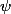on, then we for each linear map, there is a unique adjoint mapsatisfyingWe can constructexplicitly by lettingbe the mapsending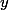toand thenThis defines an anti-automorphism of the matrix algebra: that is, a linear bijectionwhich reverses the order of multiplication. For example, ifandis the standard symmetric bilinear form thenis matrix transposition.

Theorem. The map sending a bilinear form to its adjoint anti-automorphism is a bijection between

1. equivalence classes of nonsingular bilinear forms on, up to multiplication by scalars; and
2. anti-automorphisms of.

The main thing to be proved is that every anti-automorphism comes from some bilinear form. This relies on the Skolem-Noether theorem, that every automorphism ofis conjugation by some invertible matrix.

We say that an anti-automorphism is an involution if its square is the identity. Under the bijection, a bilinear form corresponds to an involution if and only if it is either symmetric or alternating.

### The Rosati involution

Let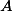be an abelian variety. Recall that a polarisation is an isogenyassociated with an ample line bundle. This should be thought of as being "like a bilinear form on" although no such thing exists directly. Being "associated with a line bundle" implies the symmetry condition, and the ampleness is a positivity condition.

We can therefore apply the same formula as in linear algebra to define an involution of the endomorphism algebra: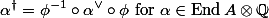whereis the inverse ofin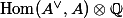. This is called the Rosati involution associated with the polarisation. (Note that the involution depends on the choice of polarisation. It preserves the order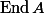if the polarisation is principal, but not necessarily otherwise.)

If we look at homology, either the-adic Tate modules over any field or the-Hodge structure over the complex numbers, then polarisations give rise to symplectic forms (Weil pairings or Riemann forms). The Rosati involution satisfies the adjoint identity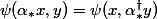for these symplectic forms, and also for the Hermitian form on the tangent space of a complex abelian variety.

### Positivity of the Rosati involution

Let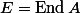. For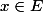, we define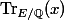to be the trace of the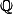-linear map.

The Rosati involution is a positive involution, meaning thatThe justification for this definition is that an involution ofis positive if and only if its corresponding bilinear form onis either positive or negative definite. The "or negative definite" might seem a bit odd, but it is because involutions only correspond to bilinear forms up to multiplication by a scalar; in particular we can multiply the form by -1.

Hence the positivity of the Rosati involution says that if we think of the polarisation as being "like a bilinear form", then it is "positive or negative definite" (but -1 times a polarisation is not a polarisation, so we can treat polarisations as always being "positive definite").

The general proof that the Rosati involution is positive (valid over all fields, including those of positive characteristic) uses étale cohomology to show thatis equal to an intersection number involving ample divisors. I shall give a simpler proof over the complex numbers only, using the positive-definiteness of the Hermitian form associated with a polarisation.

Letbe the tangent spaceand recall that a polarisation induces a positive-definite Hermitian formon. Anyinduces a linear map, and just as for the symplectic Riemann form,is the adjoint involution with respect to.

Lemma. If, then all eigenvalues ofonare nonnegative real numbers, and at least one is positive.

Proof. Letbe an eigenvector ofwith eigenvalue. ThenBecauseis positive definite,is a nonnegative real number and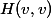is a positive real number, sois a nonnegative real number.

Now consideracting onby left multiplication. The eigenvalues ofonare simply its eigenvalues on, repeatedtimes.

Finallyembeds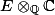as a subspace ofwhich is stable under left multiplication by. The eigenvalues ofacting on this subspace must be a subset of its eigenvalues on, so are all nonnegative real numbers.

We note also that. This is because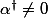so there is somesuch that. Thenso.

The trace ofis the sum of its eigenvalues on. We have shown that these are all nonnegative, and becaue, at least one eigenvalue is nonzero. Hence the sum of the eigenvalues is positive.

1. Endomorphisms of simple abelian varieties From Martin's Blog

Today I will discuss the classification of endomorphism algebras of simple abelian varieties. The endomorphism algebra of a non-simple abelian variety can easily be computed from the endomorphism algebras of its simple factors. For a simple abelia...